# 计算统计学与数据科学 02：统计学习

## 墨尔本大学 MAST90083 课程笔记

Posted by YEY on August 10, 2020

# Lecture 02 统计学习

• Gareth, J., Daniela, W., Trevor, H., & Robert, T. (2013). An intruduction to statistical learning: with applications in R. Spinger.
• Hastie, T., Tibshirani, R., & Friedman, J. (2009). The elements of statistical learning: data mining, inference, and prediction. Spinger Science & Business Media.

## 1. 什么是统计学习

### 1.1 案例学习

#### 例子1：广告预算与产品销量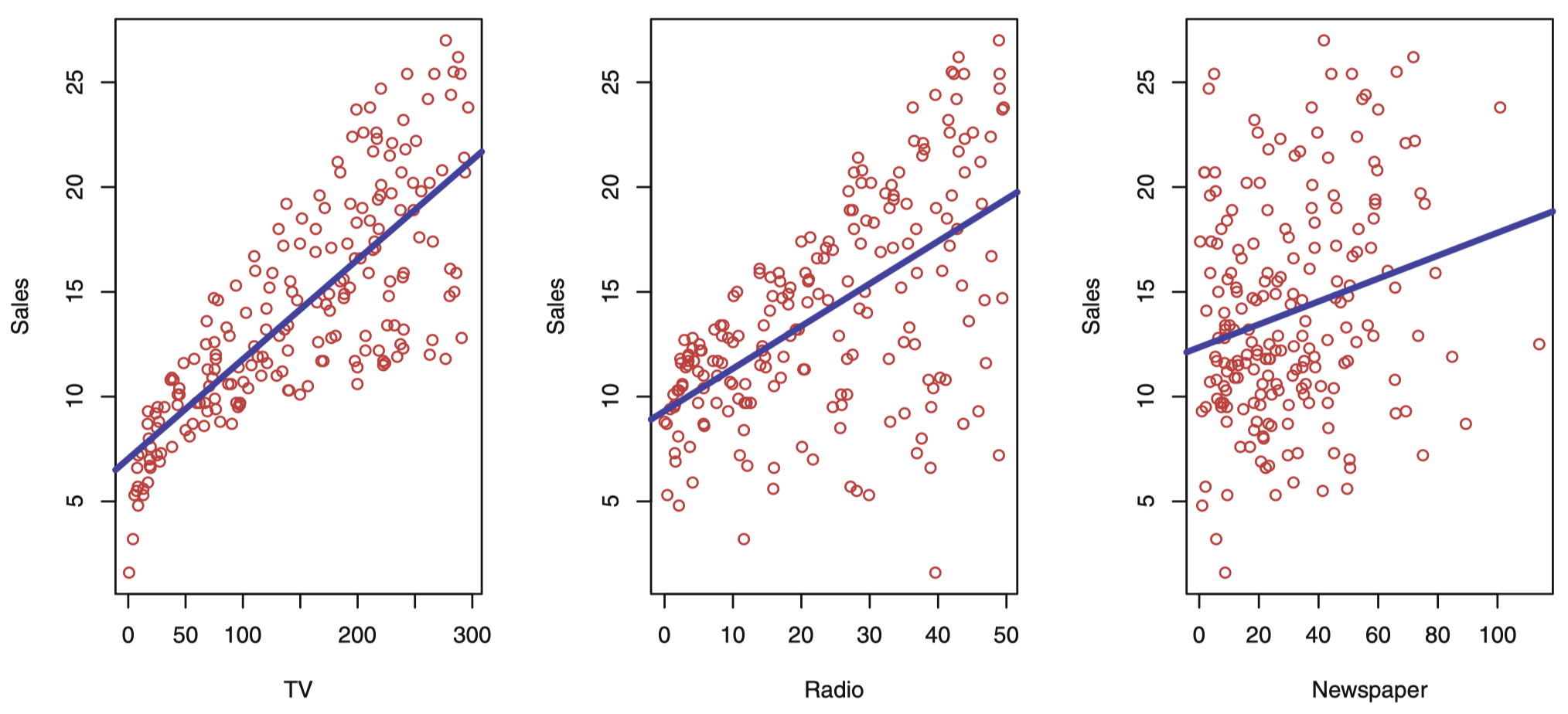$Y=f(X)+\epsilon$

#### 例子2：个人收入与受教育年限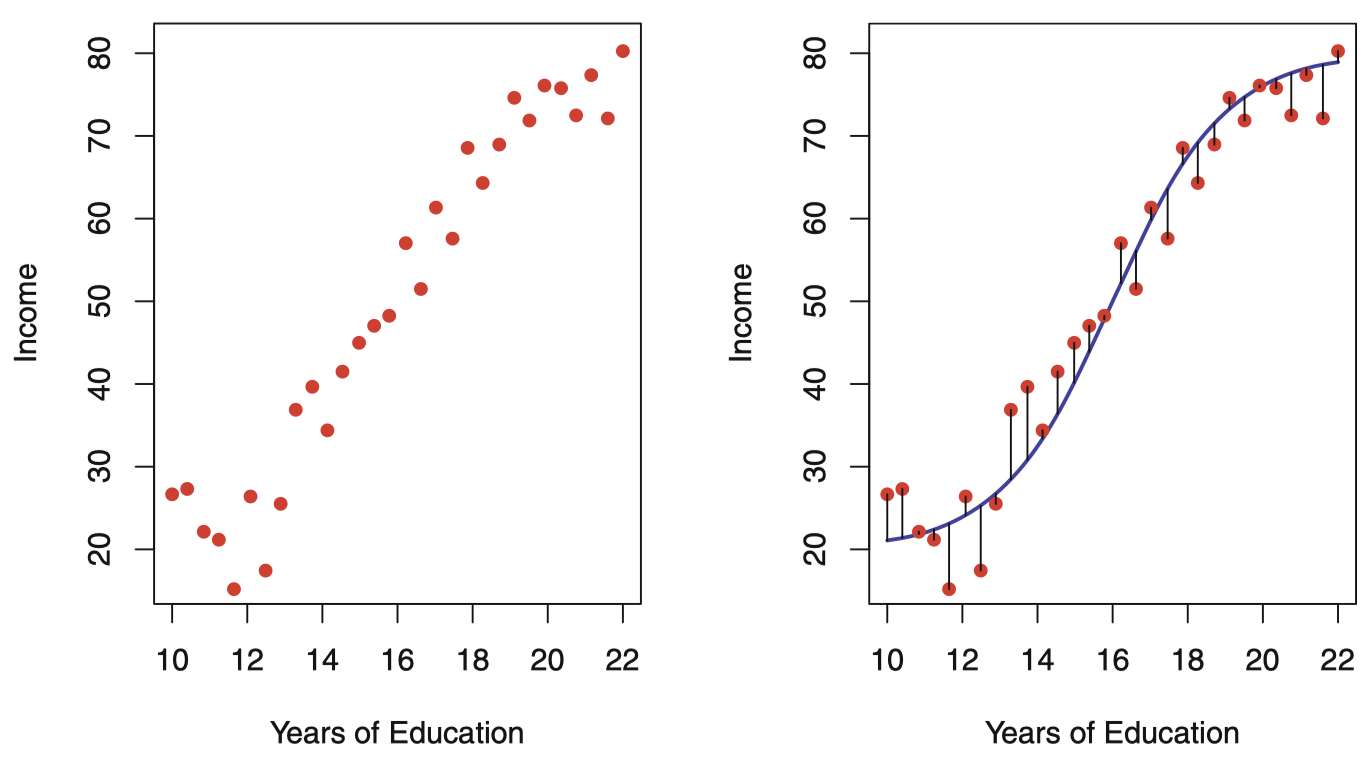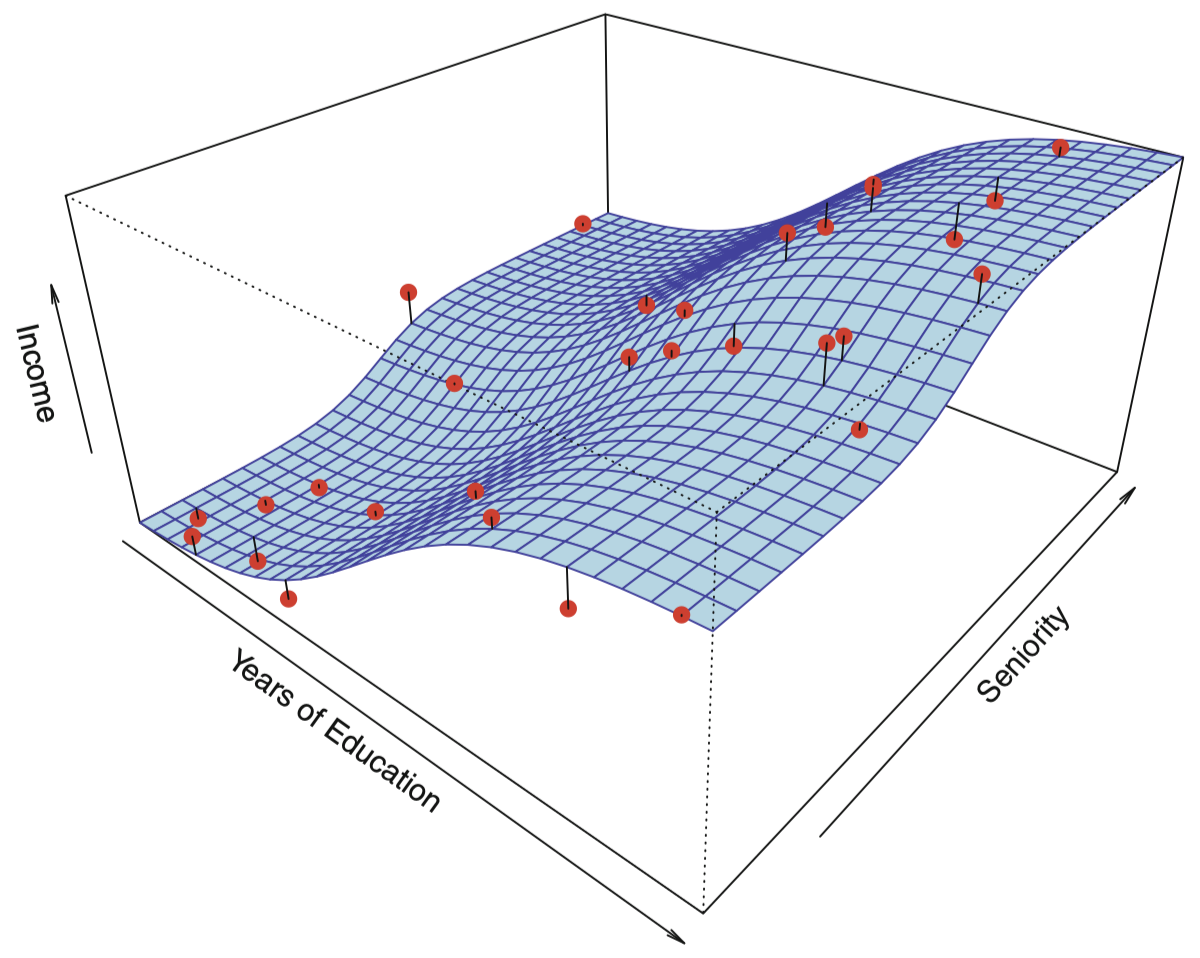### 1.2 为什么需要估计 $f$

#### 预测

$\hat Y=\hat f(X)$

$\hat Y$ 作为响应变量 $Y$ 的预测，其准确性依赖于两个量：可减小误差 (reducible error)不可减小误差 (irreducible error)。通常，$\hat f$ 并不是 $f$ 的一个完美估计，这种不准确性也会引入一些误差，但这类误差是 可减小的，因为我们实际上有能力提高 $f$ 的准确性，只要选择更合适的统计学习技术去估计 $f$ 就可能降低这种误差。然而，即使有可能构造出一个 $f$ 的完美估计，使得我们的估计响应取得 $\hat Y=f(X)$ 的形式，我们的预测中仍然会存在一些误差。这是因为 $Y$ 还是一个关于 $\epsilon$ 的函数，并且按照定义，$\epsilon$ 无法通过 $X$ 来预测。因此，与 $\epsilon$ 相关的可变性同样影响着我们预测的准确性。这部分误差就被称为 不可减小 误差，因为 无论我们对 $f$ 估计得多么好，我们都无法减小由 $\epsilon$ 引入的误差

\begin{align} E(Y-\hat Y)^2 &= E[\,f(X)+\epsilon -\hat f(X)]^2 \\ &= \underbrace{[\,f(X)-\hat f(X)]^2}_{可减小误差} + \underbrace{\mathrm{Var}(\epsilon)}_{不可减小误差} \end{align}

• 首先，$\epsilon$ 可能包含了某些 未测量变量 (unmeasured variables)，它们对于预测 $Y$ 有用：由于没有测量它们，所以 $f$ 在预测时无法使用这些变量。
• 其次，$\epsilon$ 可能还包含了一些 不可测量的差异 (unmeasurable variation)。例如，某天某个特定患者对于某种药物的不良反应风险可能会有所不同，具体取决于药物本身在制造过程中产生的差异，或者患者当天的情绪状态等。

#### 推断

• 哪些预测变量与响应变量相关？ 通常情况下用于预测的变量中只有一小部分与 $Y$ 充分相关，从一大组可能的变量中根据应用的需要识别一些重要的预测变量是极其有必要的。

• 响应变量与每个预测变量之间的关系是什么？ 一些预测变量与 $Y$ 正相关，这意味着，当增加相应的预测变量的值时，$Y$ 的值也会增加。而另一些预测变量则与 $Y$ 呈 负相关。取决于 $f$ 的复杂性，响应变量与某个给定的预测变量之间的关系也可能依赖于其他预测变量的值。

• $Y$ 与每个预测变量的关系是否能用一个线性方程概括，或者需要更复杂的形式？ 过去，大多数估计 $f$ 的方法都采用线性形式。在一些情况下，这种假设是合理的甚至是比较理想的方式。但更一般的情况下，真正的关系 可能更为复杂，此时，线性模型可能无法提供一种准确的表达。

### 1.3 如何估计 $f$

#### 参数方法

1. 首先，我们对函数 $f$ 的形式或形状给出一个假设。例如，一个常用假设是 $f$ 关于 $X$ 是线性的：

$f(X)=\beta_0+\beta_1 X_1 +\beta_2 X_2 +\cdots + \beta_p X_p$

这是一个 线性模型 (linear model)，我们将在下节课展开讨论。一旦假设 $f$ 是线性的，估计 $f$ 的问题将被大大简化：我们不必去估计一个完全任意的 $p$ 维函数 $f(X)$，而只需估计 $p+1$ 个系数 $\beta_0,\beta_1,\dots,\beta_p$。

2. 在选定一个模型后，我们需要用训练数据集去 拟合训练 模型。在上面的线性模型中，我们需要估计参数 $\beta_0,\beta_1,\dots,\beta_p$。这就是说，我们希望找到这些参数的值使得：

$Y\approx \beta_0+\beta_1 X_1 +\beta_2 X_2 +\cdots + \beta_p X_p$

对于该模型，最常用的拟合方法称是 普通最小二乘法 (ordinary least squares, OLS)，具体细节将在下节课中讨论。然而，最小二乘法只是众多用于拟合线性模型的方法中的一种，在后面的课程中，我们会讨论一些其他用来估计该模型参数的方法。

$\texttt {income} \approx \beta_0+\beta_1 \times \texttt{education}+\beta_2 \times \texttt{seniority}$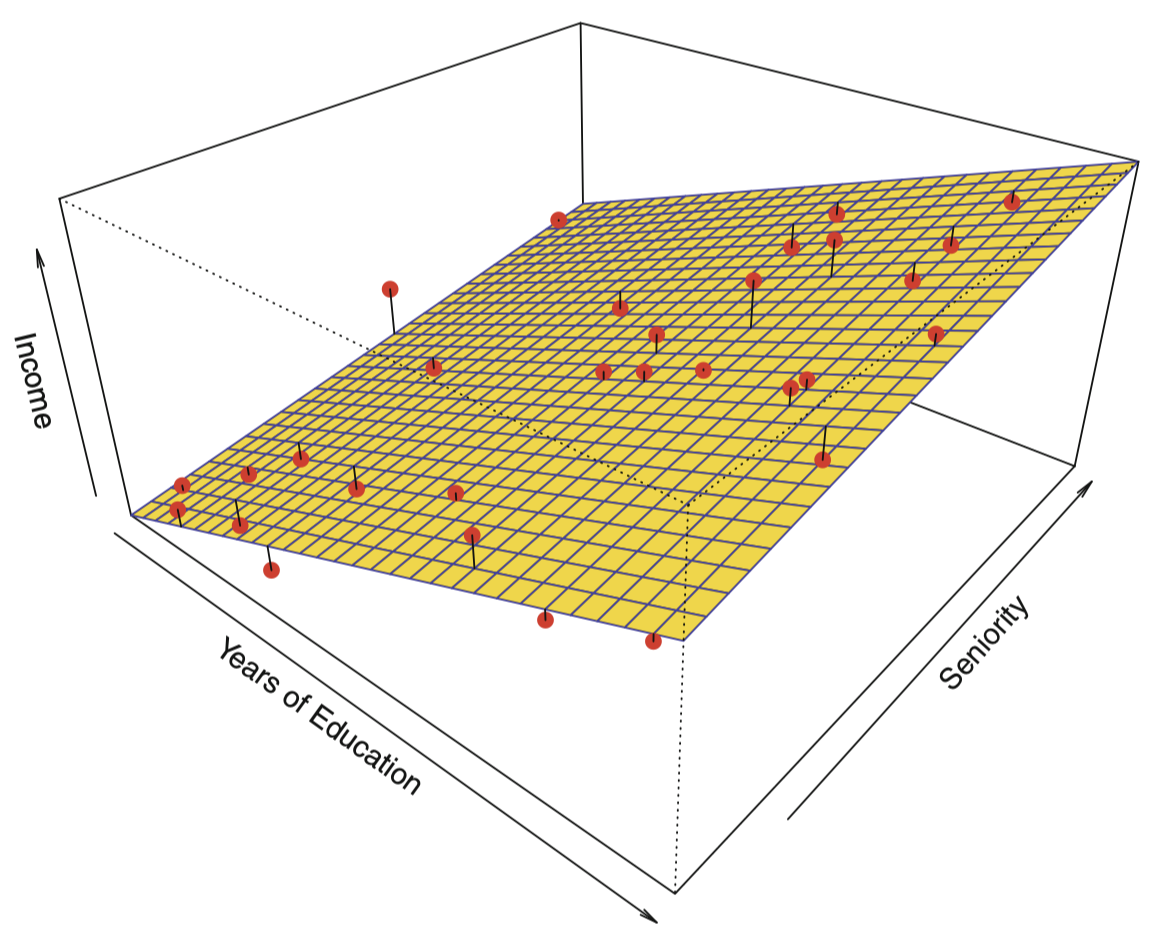#### 非参数方法

• 非参数方法不需要对函数 $f$ 的形式事先做明确的假设。
• 非参数方法的优点：不限定函数 $f$ 的具体形式，可以在更大范围内选择更适宜 $f$ 形状的估计。
• 非参数方法的主要缺点：由于没有将估计 $f$ 的问题简化为估计一组参数，因此，为了获得一个关于 $f$ 的准确估计，需要大量的观测数据 (通常远超参数方法所需要的数据量)。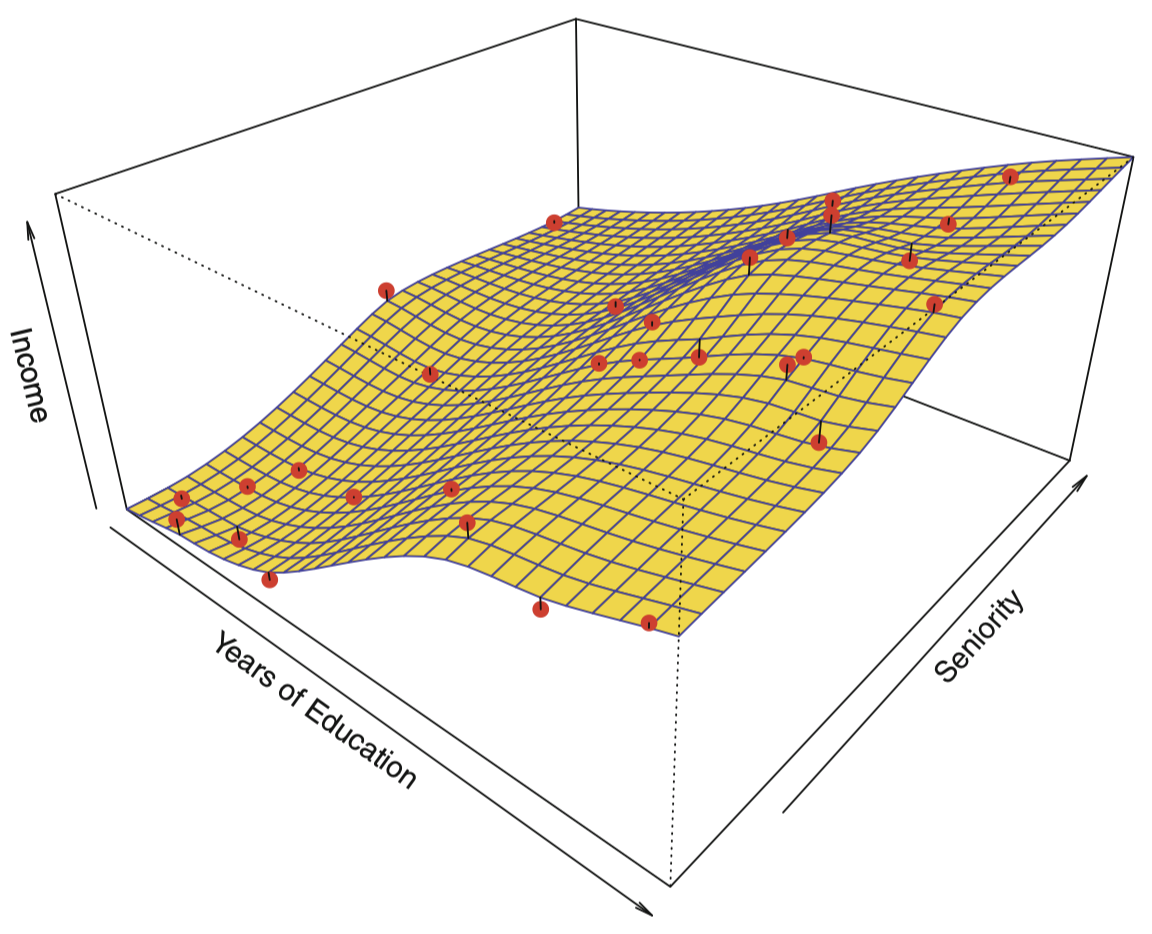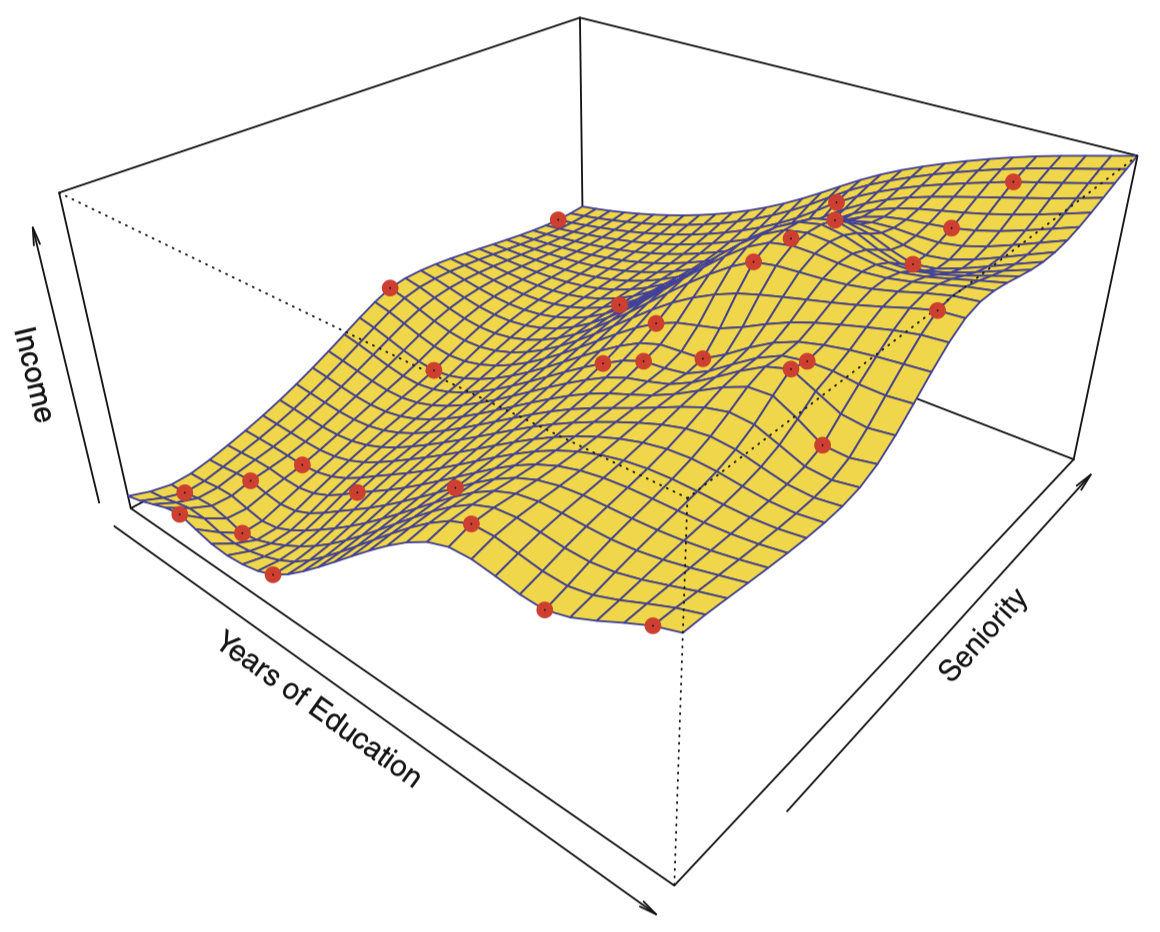### 1.4 预测准确率和模型解释性的权衡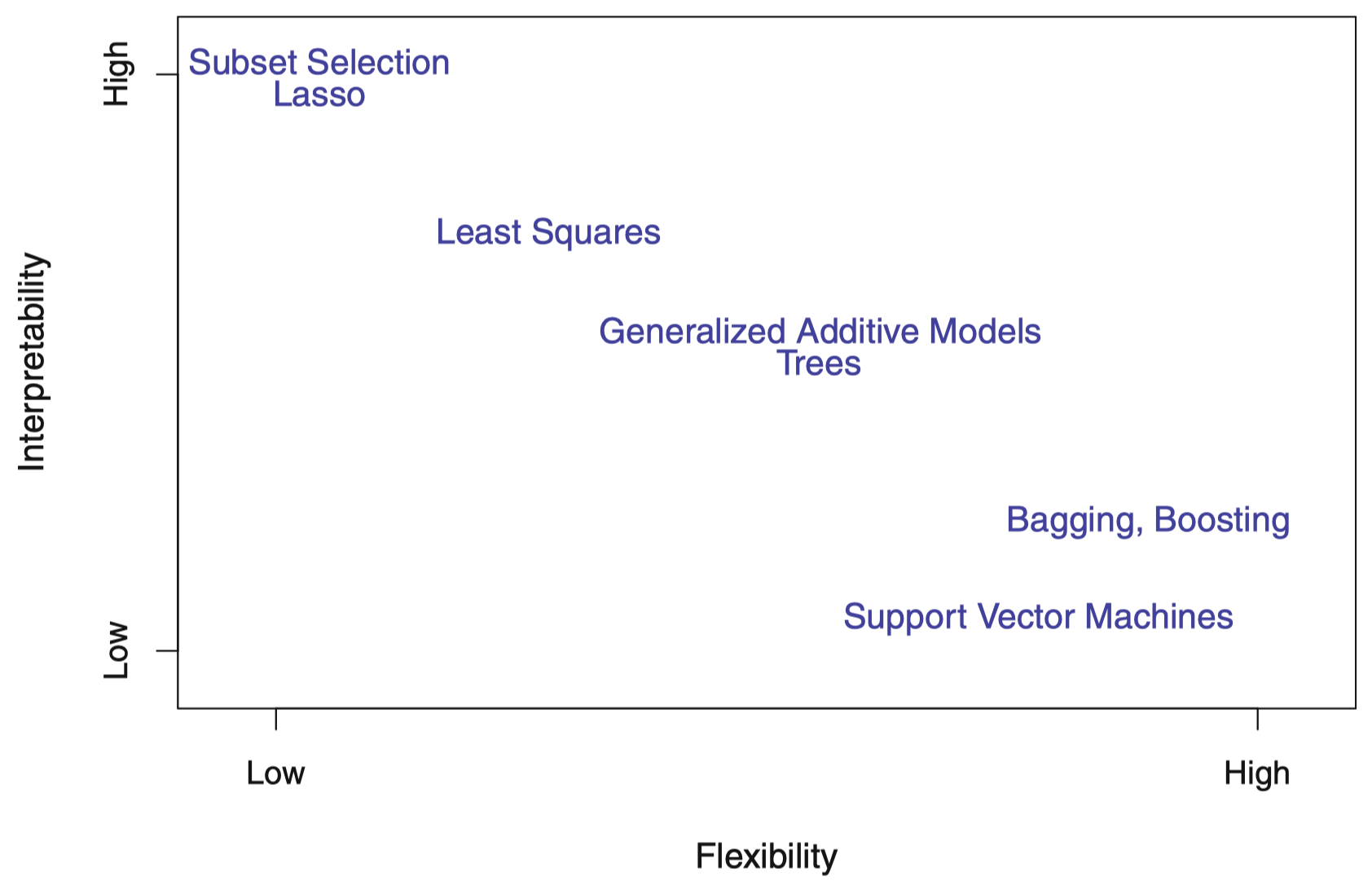### 1.5 监督学习 VS. 无监督学习

#### 无监督学习## 2. 评价模型准确率

### 2.1 拟合效果检验

$\mathrm{MSE}=\dfrac{1}{n}\sum_{i=1}^{n}(y_i-\hat f(x_i))^2$

$\mathrm{Ave} (y_0-\hat f(x_0))^2$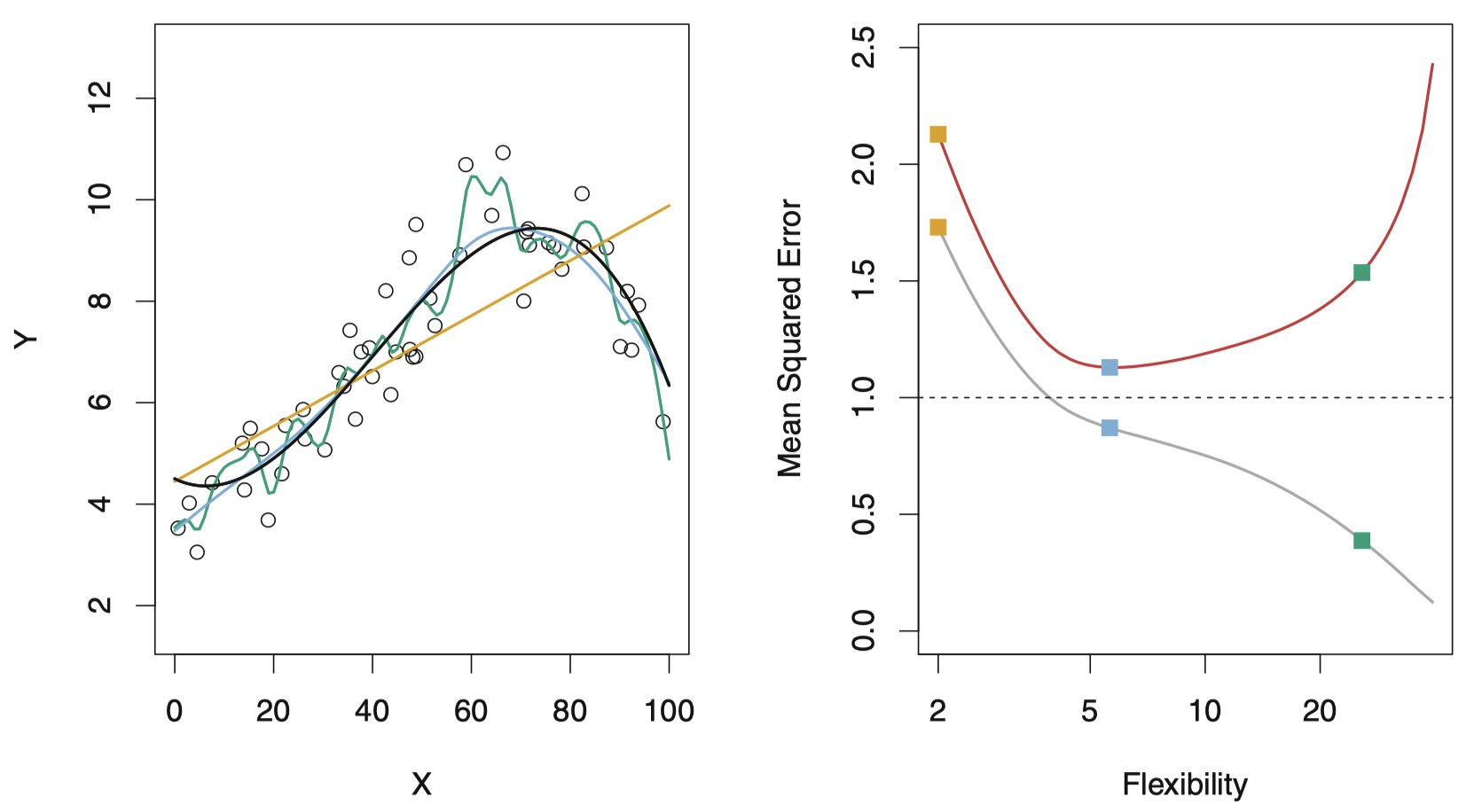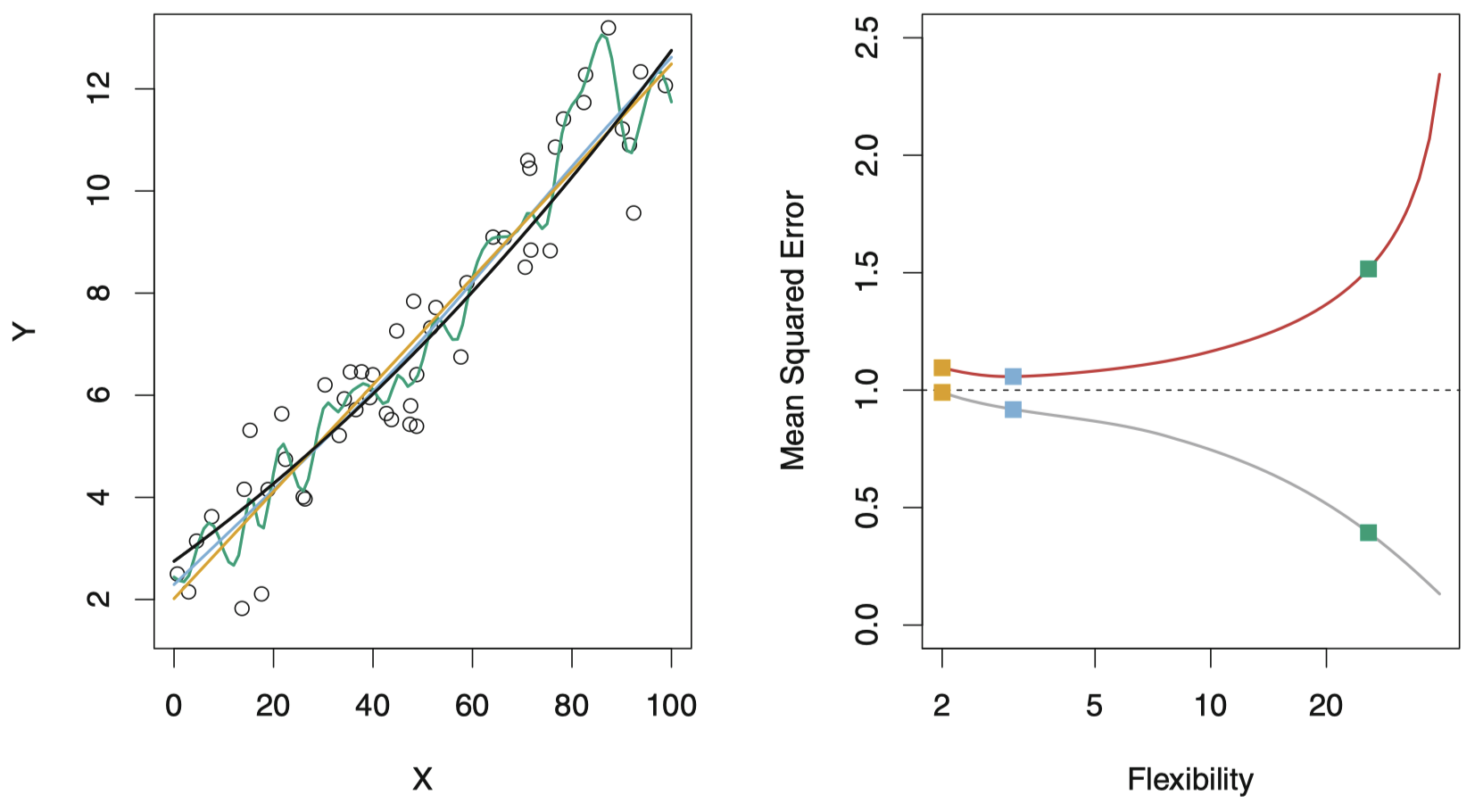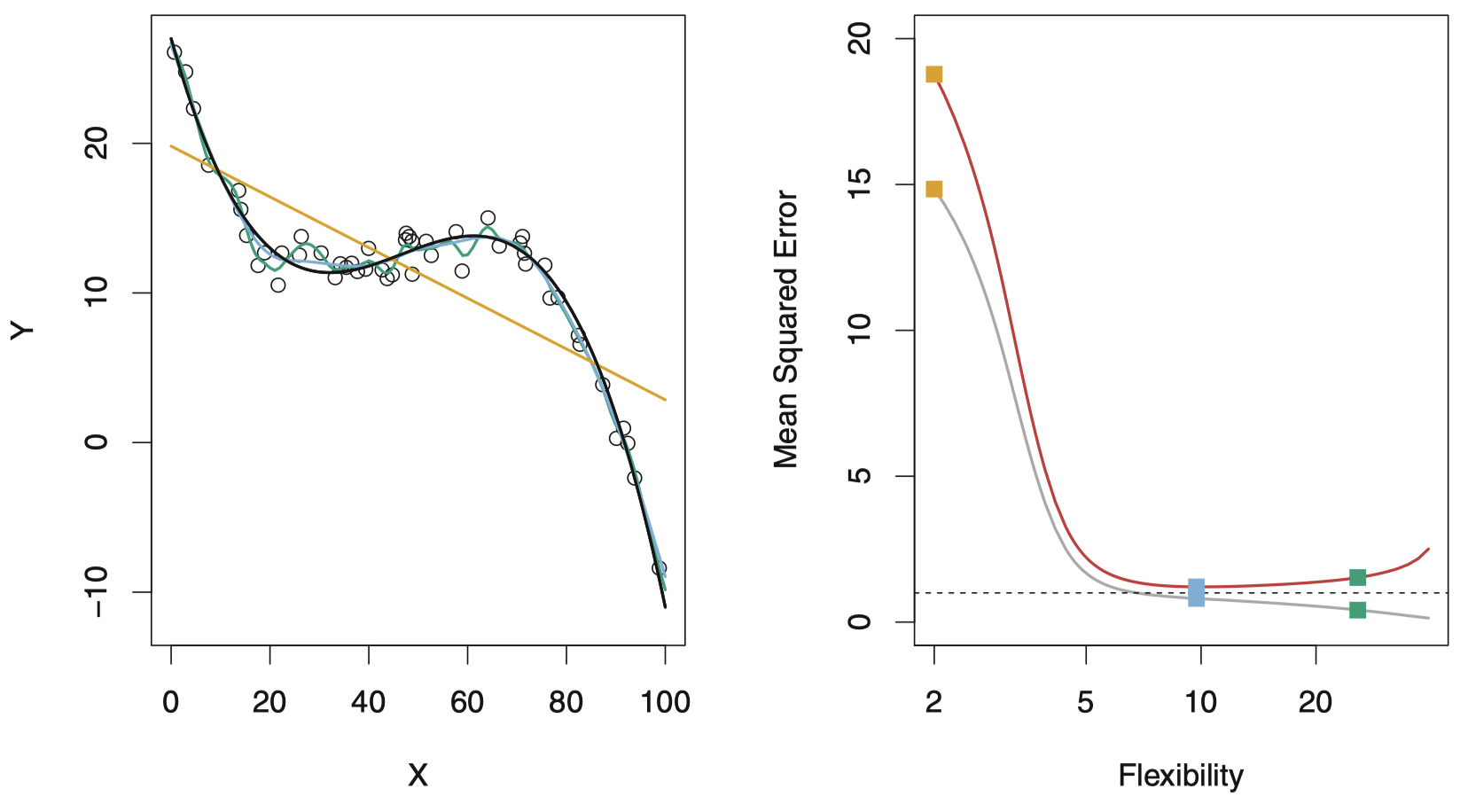### 2.2 偏差-方差权衡

$E\left(y_0 - \hat f(x_0)\right)^2=\mathrm{Var}(\hat f(x_0))+[\mathrm{Bias}(\hat f(x_0))]^2 + \mathrm{Var}(\epsilon)$

• 方差 是指如果使用不同的训练数据集，所估计的 $\hat f$ 的变化量。如果一个模型有较大的方差，那么训练数据集微小的变化则会导致 $\hat f$ 较大的改变。通常，灵活度越高的统计模型具有更高的方差。

• 偏差 是指通过一个简单模型去逼近真实函数的过程中引入的误差，其构成可能非常复杂。通常，灵活度越高的方法所产生的偏差越小。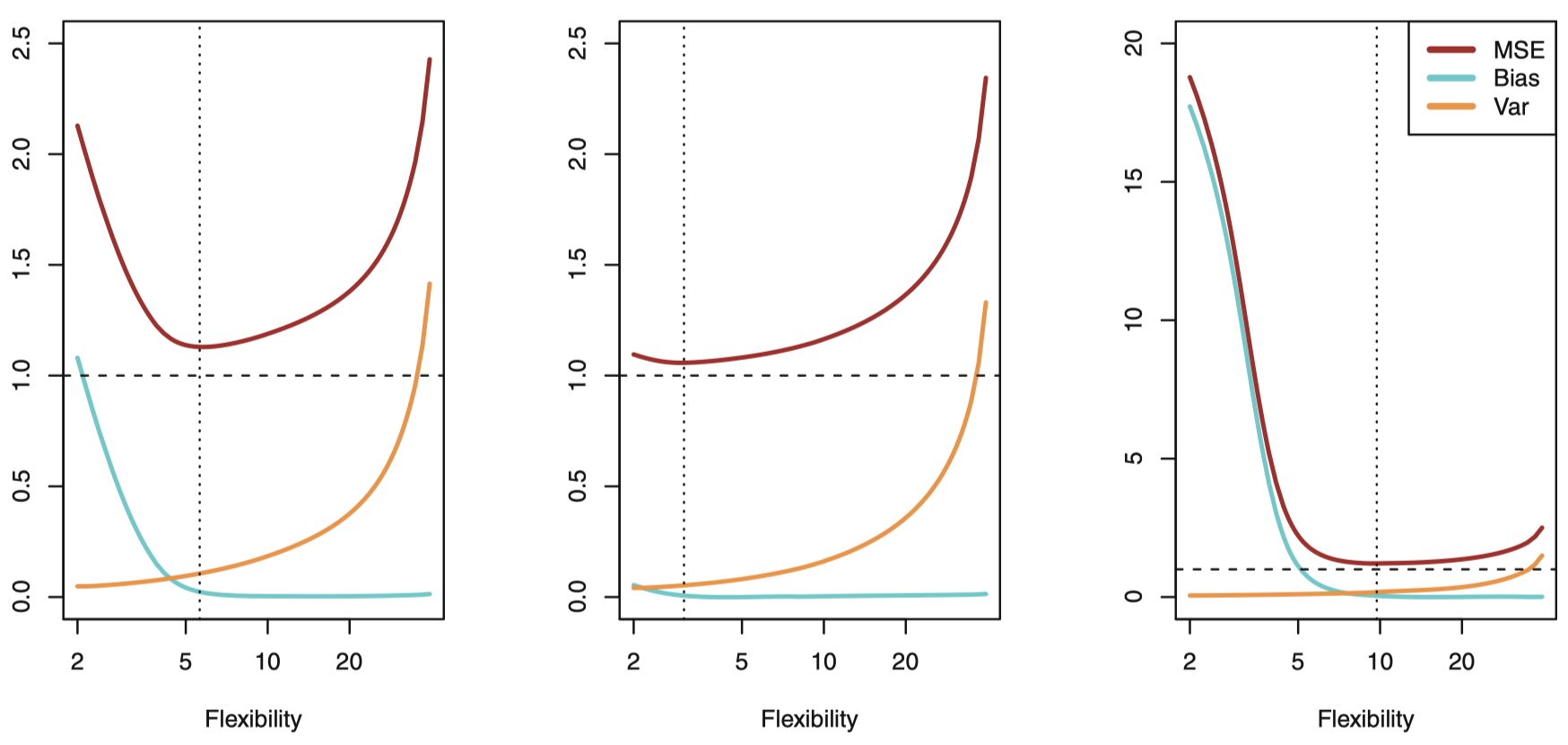### 2.3 分类模型

$\dfrac{1}{n}\sum_{i=1}^{n}I(y_i \ne \hat y_i)$

$\mathrm{Ave}(I(y_0 \ne \hat y_0))$

#### 贝叶斯分类器

$\Pr (Y=j\mid X=x_0)$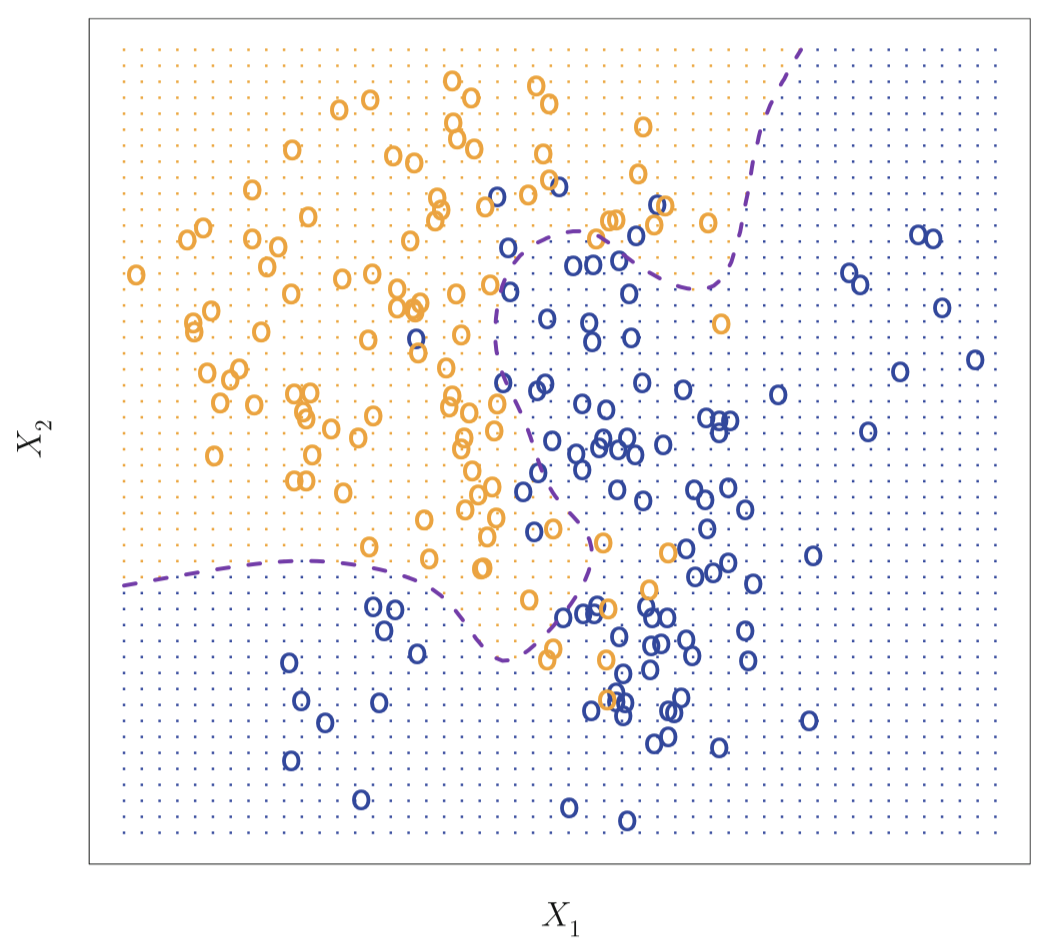$1-E\left(\max_j \Pr(Y=j\mid X)\right)$

#### K 最近邻方法

$\Pr(Y=j\mid X=x_0)=\dfrac{1}{K}\sum_{i\in \mathcal N_0} I(y_i=j)$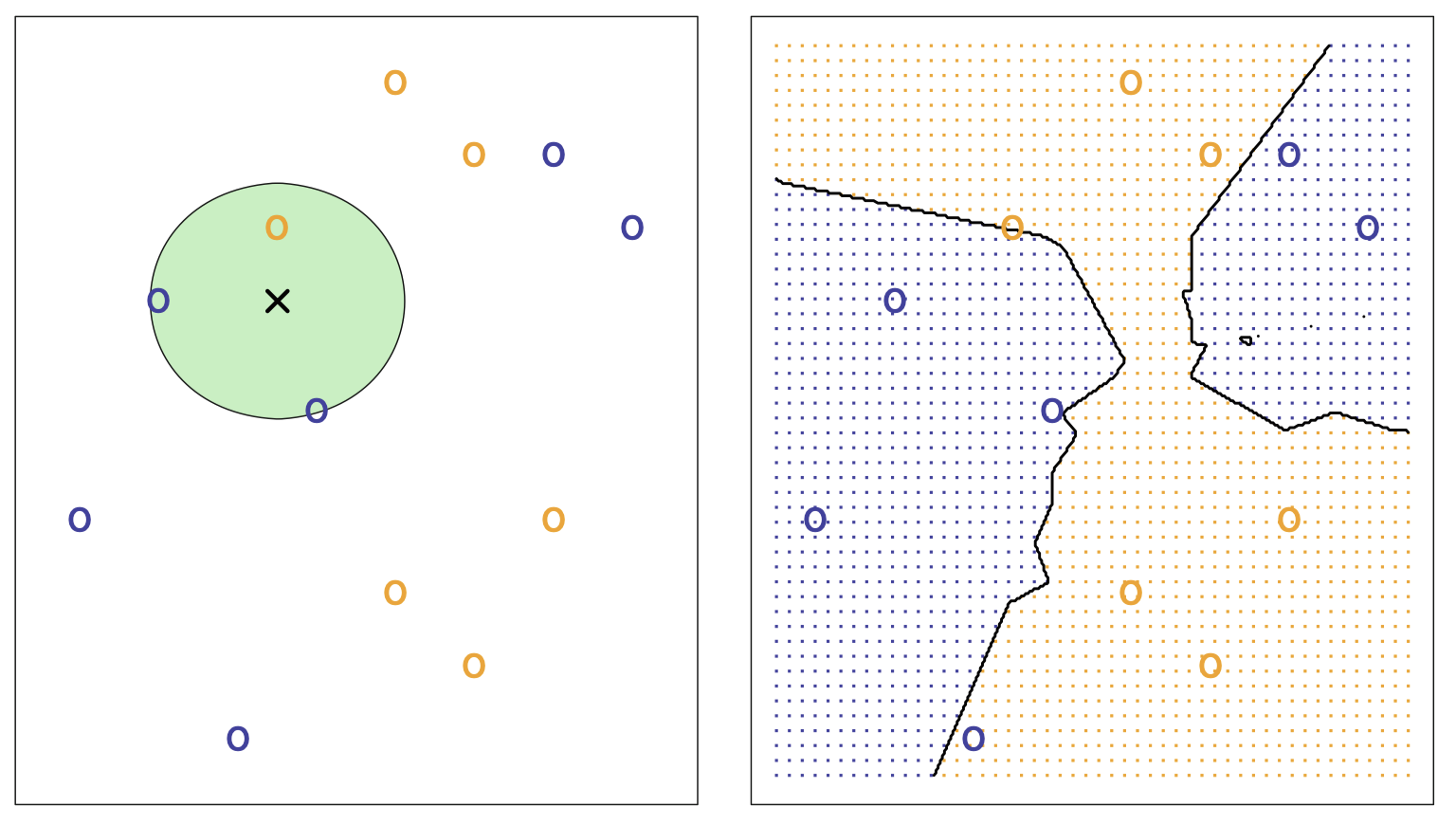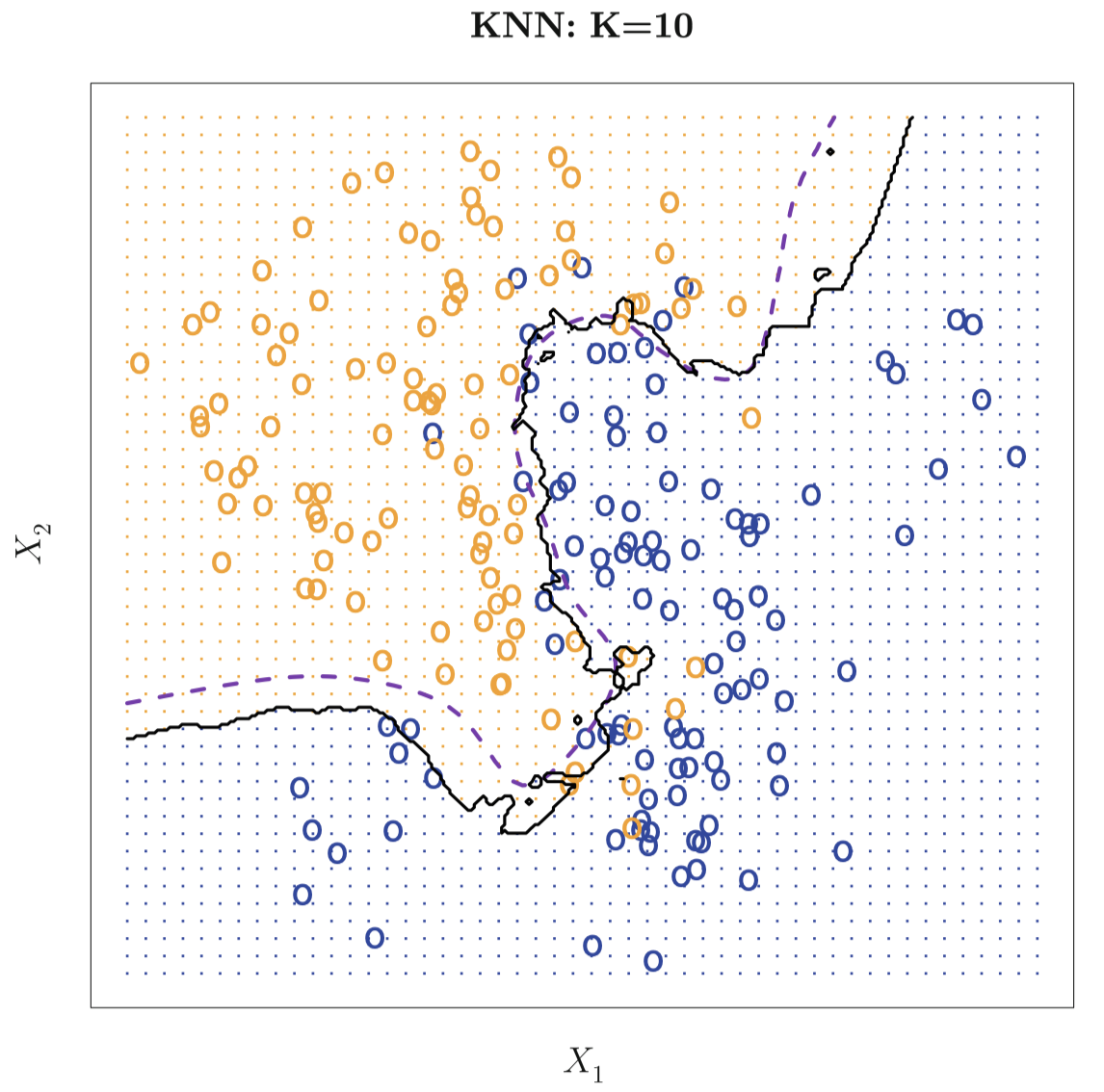$K$ 的选择对 KNN 分类器的性能具有决定性影响。图 16 展示了对同一数据集应用不同 $K$ 值的情况：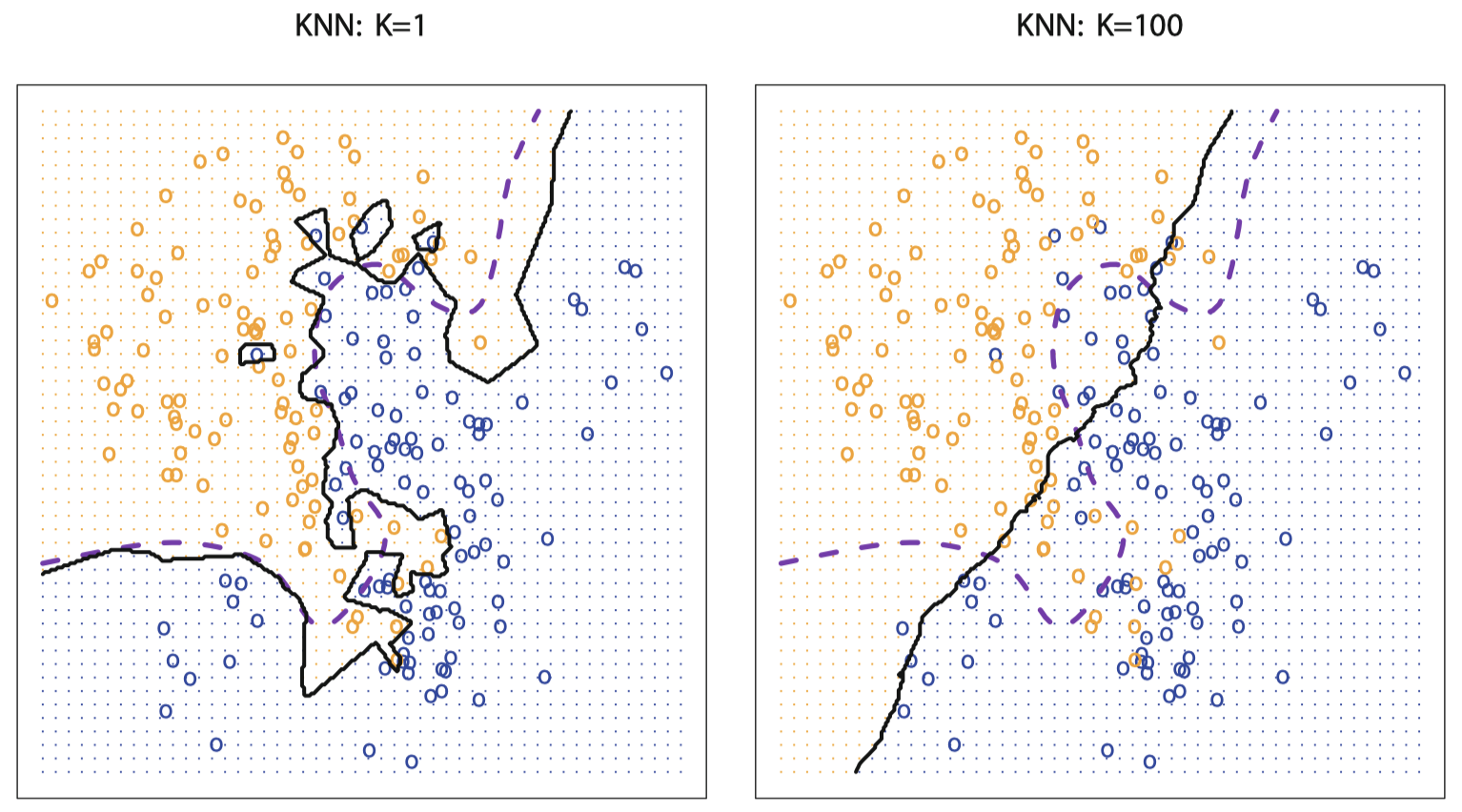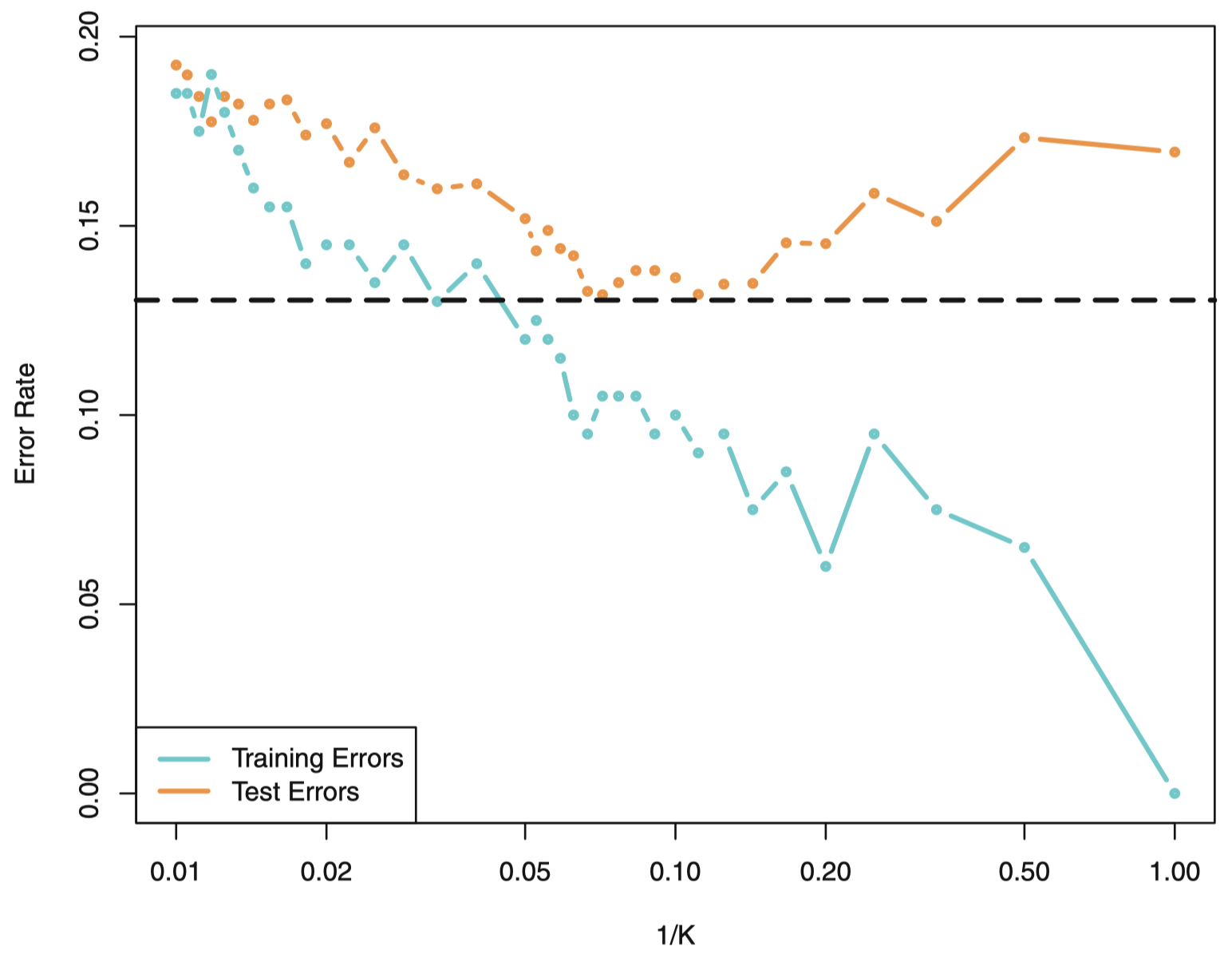## 3. 思考

(a) 样本量 $n$ 非常大，预测变量 $p$ 的数量很少。

(b) 预测变量 $p$ 的数量非常多，而观测数量 $n$ 很少。

(c) 预测变量与响应之间的关系是高度非线性的。

(d) 误差项的方差即 $\sigma^2=\mathrm{Var}(\epsilon)$ 非常大。本作品采用知识共享署名-非商业性使用-相同方式共享 4.0 国际许可协议进行许可。 欢迎转载，并请注明来自：YEY 的博客 同时保持文章内容的完整和以上声明信息！